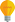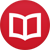# What does it mean for a function to be well-defined?

by Jakub MarianTip: See my list of theMost Common Mistakes in English. It will teach you how to avoid mis­takes with com­mas, pre­pos­i­tions, ir­reg­u­lar verbs, and much more.

A common addendum to a formula defining a function in mathematical texts is, “it remains to be shown that the function is well defined.” For many beginning students of mathematics and technical fields, the reason why we sometimes have to check “well-definedness” while in other cases we don’t remains elusive.

The truth is, calling something “well-defined” is kind of a misnomer. Mathematicians define mathematical objects, and if the definition is syntactically correct, the objects are always “well-defined”. What may happen, however, is that the defined object is not a function.

To understand the notion of “well-definedness”, we first have to take a look at objects more general than functions, namely binary relations. A binary relation is simply a set of pairs. For example, $R = \{(1,2),(2,3)\}$ is a relation. A relation tells us whether two objects are related; for instance numbers $1$ and $2$ are related with respect to $R$, which we write as $1\,R\,2$.

This looks weird, but as soon as I tell you that “$<$” is an example of a relation, the notation $1 < 2$ starts looking quite acceptable, doesn't it? Formally, “$<$” is just the set $\{(1,2),(1,3),(2,3),...\}$ of all pairs of numbers such that the first one is smaller than the second one.

But, what does it have to do with functions? It turns out that a function is just a special case of a relation. A relation $f$ is called a function if

$$\text{for each } x \text{ there is at most one } y \text{ such that } x\,f\,y \text{ (i.e. }(x,y) ∈ f\text{)}$$

This one $y$ is called the value of $f$ at $x$ and denoted $f(x)$. For example, since $\cos(0) = 1$, $1$ is the only number such that

$$0\,{\cos}\,1$$

which could be read as “$0$ goes to $1$ through $\cos$” and is just another way of writing

$$(0,1) ∈ \cos$$ How can a relation fail to be a function? “$<$” is not a function because, for instance, $1<2$ and $1<3$, so there is not a unique candidate for ${<}(1)$.

## Well-definedness

What often happens in mathematics is that the way we define an object leads to a relation which may or may not be a function. For example, imagine you try to define

$$f(x/y) = x+y,\quad x, y ∈ ℕ$$

Strictly speaking, this is not a syntactically correct definition because it uses the notation $f(…)$ for an object that will turn out not to be a function. What this expression is really supposed to mean is that we define a relation:

$$f = \{(x/y,x+y): x,y ∈ ℕ\}$$

Now we can ask: Is $f$ a function? The answer is “no” because, for instance, $(1,2) ∈ f$ (for $x = y = 1$) as well as $(1,4) ∈ f$ (for $x = y = 2$), so there is not a unique candidate for $f(1)$.

In mathematical jargon that developed long before our current understanding of functions as sets of pairs, we refer to a relation whose being a function requires a proof as well-defined. Instead of defining a relation and checking its “functionality”, we just write it down as if we knew it was a function and check whether everything makes sense.

For example, in the case of our $f$ above, we would write:

$$f(1) = f(1/1) = 1+1 = 2\\ f(1) = f(2/2) = 2+2 = 4$$

which is not possible, so $f$ is not well-defined. Mathematically, the above expression is nonsense. You just have to keep in mind that what is really happening is checking whether the relation $f$ is a function, as described above.

## When do we have to prove that a function is well-defined?

The answer suggests itself: When it is not obvious that it is. For example, when you define $f(x) = \sin(x)\cos(x)$, it is obvious that $f$ is a function because we just assigned exactly one value to each point. On the other hand, let $g$ be defined (for whatever reason) as

$$g(x/y) = \sqrt[y]{e^x}+\log(x)-\log(y),\quad x,y > 0$$

Is it obviously a function? Some people would say it requires a proof, so we would go

$$g(x/y) = (e^x)^{÷{1}{y}} +\log(x/y) = e^{x/y}+\log(x/y)$$

Substituting $z = x/y$, we get that for each possible value of $z$,

$$g(z) = e^z +\log(z)$$

Therefore for each value of the argument, we get exactly one value of $g$, so $g$ is well-defined.

By the way, I have written several educational ebooks. If you get a copy, you can learn new things and support this website at the same time—why don’t you check them out?